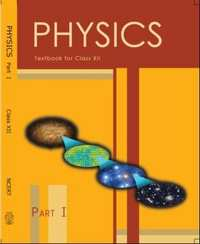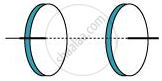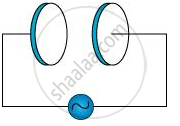Share

NCERT solutions for Class 12 Physics chapter 8 - Electromagnetic Waves

Physics Textbook for Class 12

NCERT Physics Class 12Chapter 8: Electromagnetic Waves

Chapter 8: Electromagnetic Waves solutions [Pages 286 - 287]

Q 1 | Page 286

Figure 8.6 shows a capacitor made of two circular plates each of radius 12 cm, and separated by 5.0 cm. The capacitor is being charged by an external source (not shown in the figure). The charging current is constant and equal to 0.15 A.

(a) Calculate the capacitance and the rate of charge of potential difference between the plates.

(b) Obtain the displacement current across the plates.Q 2 | Page 286

A parallel plate capacitor (Fig. 8.7) made of circular plates each of radius = 6.0 cm has a capacitance = 100 pF. The capacitor is connected to a 230 V ac supply with a (angular) frequency of 300 rad s−1.

(a) What is the rms value of the conduction current?

(b) Is the conduction current equal to the displacement current?

(c) Determine the amplitude of at a point 3.0 cm from the axis between the plates.Q 3 | Page 286

What physical quantity is the same for X-rays of wavelength 10−10 m, red light of wavelength 6800 Å and radiowaves of wavelength 500 m?

Q 4 | Page 286

A plane electromagnetic wave travels in vacuum along z-direction. What can you say about the directions of its electric and magnetic field vectors? If the frequency of the wave is 30 MHz, what is its wavelength?

Q 5 | Page 286

A radio can tune in to any station in the 7.5 MHz to 12 MHz band. What is the corresponding wavelength band?

Q 6 | Page 286

A charged particle oscillates about its mean equilibrium position with a frequency of 109 Hz. What is the frequency of the electromagnetic waves produced by the oscillator?

Q 7 | Page 286

The amplitude of the magnetic field part of a harmonic electromagnetic wave in vacuum is B0 = 510 nT. What is the amplitude of the electric field part of the wave?

Q 8 | Page 286

Suppose that the electric field amplitude of an electromagnetic wave is E0 = 120 N/C and that its frequency is ν = 50.0 MHz. (a) Determine, B0ω, k, and λ. (b) Find expressions for and B.

Q 9 | Page 286

The terminology of different parts of the electromagnetic spectrum is given in the text. Use the formula hν (for energy of a quantum of radiation: photon) and obtain the photon energy in units of eV for different parts of the electromagnetic spectrum. In what way are the different scales of photon energies that you obtain related to the sources of electromagnetic radiation?

Q 10 | Page 286

In a plane electromagnetic wave, the electric field oscillates sinusoidally at a frequency of 2.0 × 1010 Hz and amplitude 48 V m−1.

(a) What is the wavelength of the wave?

(b) What is the amplitude of the oscillating magnetic field?

(c) Show that the average energy density of the field equals the average energy density of the field. [= 3 × 108 m s−1.]

Q 11 | Page 287

Suppose that the electric field part of an electromagnetic wave in vacuum is

vecE=3.1N/C cos[(1.8 (rad)/m)y+(5.4xx10^8 (rad)/s)t]hati

(a) What is the direction of propagation?

(b) What is the wavelength λ?

(c) What is the frequency ν?

(d) What is the amplitude of the magnetic field part of the wave?

(e) Write an expression for the magnetic field part of the wave.

Q 12 | Page 287

About 5% of the power of a 100 W light bulb is converted to visible radiation. What is the average intensity of visible radiation

(a) at a distance of 1 m from the bulb?

(b) at a distance of 10 m?

Assume that the radiation is emitted isotropically and neglect reflection.

Q 13 | Page 287

Use the formula λm T= 0.29 cm K to obtain the characteristic temperature ranges for different parts of the electromagnetic spectrum. What do the numbers that you obtain tell you?

Q 14 | Page 287

Given below are some famous numbers associated with electromagnetic radiations in different contexts in physics. State the part of the electromagnetic spectrum to which each belongs.

(a) 21 cm (wavelength emitted by atomic hydrogen in interstellar space).

(b) 1057 MHz (frequency of radiation arising from two close energy levels in hydrogen; known as Lamb shift).

(c) 2.7 K [temperature associated with the isotropic radiation filling all space-thought to be a relic of the ‘big-bang’ origin of the universe].

(d) 5890 Å - 5896 Å [double lines of sodium]

(e) 14.4 keV [energy of a particular transition in 57Fe nucleus associated with a famous high resolution spectroscopic method

(Mössbauer spectroscopy)].

Q 15.1 | Page 287

Long distance radio broadcasts use short-wave bands. Why?

Q 15.2 | Page 287

It is necessary to use satellites for long distance TV transmission. Why?

Q 15.3 | Page 287

Optical and radio telescopes are built on the ground but X-ray astronomy is possible only from satellites orbiting the earth. Why?

Q 15.4 | Page 287

The small ozone layer on top of the stratosphere is crucial for human survival. Why?

Q 15.5 | Page 287

If the earth did not have an atmosphere, would its average surface temperature be higher or lower than what it is now?

Q 15.6 | Page 287

Some scientists have predicted that a global nuclear war on the earth would be followed by a severe ‘nuclear winter’ with a devastating effect on life on earth. What might be the basis of this prediction?

Chapter 8: Electromagnetic Waves

NCERT Physics Class 12NCERT solutions for Class 12 Physics chapter 8 - Electromagnetic Waves

NCERT solutions for Class 12 Physics chapter 8 (Electromagnetic Waves) include all questions with solution and detail explanation. This will clear students doubts about any question and improve application skills while preparing for board exams. The detailed, step-by-step solutions will help you understand the concepts better and clear your confusions, if any. Shaalaa.com has the CBSE Physics Textbook for Class 12 solutions in a manner that help students grasp basic concepts better and faster.

Further, we at Shaalaa.com are providing such solutions so that students can prepare for written exams. NCERT textbook solutions can be a core help for self-study and acts as a perfect self-help guidance for students.

Concepts covered in Class 12 Physics chapter 8 Electromagnetic Waves are Elementary Facts About Electromagnetic Wave Uses, Electromagnetic Spectrum, Transverse Nature of Electromagnetic Waves, Electromagnetic Waves, Displacement Current.

Using NCERT Class 12 solutions Electromagnetic Waves exercise by students are an easy way to prepare for the exams, as they involve solutions arranged chapter-wise also page wise. The questions involved in NCERT Solutions are important questions that can be asked in the final exam. Maximum students of CBSE Class 12 prefer NCERT Textbook Solutions to score more in exam.

Get the free view of chapter 8 Electromagnetic Waves Class 12 extra questions for Physics and can use Shaalaa.com to keep it handy for your exam preparation

S